## Understanding the Transaction Matching Engine

This topic helps you understand how the Transaction Matching engine evaluates transactions.

The examples in this topic assume the following transactions in Source System data source and Sub System data source.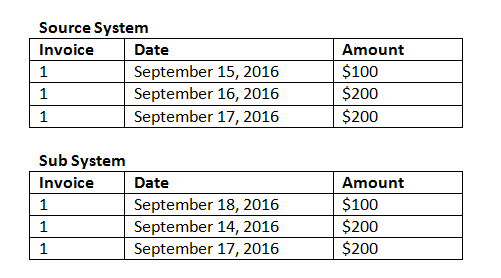Match Rules

1. Source System "Invoice" exactly matches Sub System invoice

2. Source System matches within a -1 to +3 tolerance of Sub System Date

3. Source System "Amount" exactly matches Sub System amount

The Source System and Sub System "Amount" attribute is the Balancing Attribute.

Order of Evaluation / First One Matching Wins

Transactions are evaluated for matching in the order in which the transactions exist in the database, and the first transaction evaluated for the match that satisfies the conditions will be selected for the match.

Order of Evaluation / Lowest Transaction ID Matches First

When transactions are loaded, they are assigned a Transaction ID sequentially. Therefore, the matching transaction with the lowest Transaction ID means it was loaded first and will be first to match.

In a 1 to 1 match, if two transactions exist that qualify as a match with a third transaction, but only one can be matched, the transaction with the lowest Transaction ID will be the one selected as the match.

For subset rules, matching will sort the transactions based on both amount and transaction ID during match creation. If two transactions have the same amount, the one with the lowest transaction ID will be used in the match.

Note that if you ran the same rule with No Ambiguous selected, all transactions will remain unmatched (as expected).

Evaluating Tolerances

There are three ways to set tolerance levels to allow matching of transactions that have variances. You can set:

• a tolerance variance value that is applied to the transactions. For example, auto match with up to a variance of -1 and +3.
• a tolerance threshold level expressed as a percentage. For example, auto match with up to 0.5% of the value of the amount.
• a tolerance threshold level expressed as a percentage along with a variance amount. For example, auto match with up to 1.0% of the value of the amount up to a maximum amount of 100.00.

Note:

The percentage value can not be more than 100 for both high and low tolerance and can be used for Number or Integer data source attributes.

Evaluating Tolerances Set as Values

When evaluating tolerances that are a set tolerance value, the calculation is impacted by how the high/low tolerance values are applied to transactions. For example, in the sample transactions where we apply a tolerance to the Date values, we have an authorized tolerance of -1 and +3. If you apply these values to the first record in the Source System table, then the first record in the Sub System table satisfies the match rule because September 18 is less than or equal to +3 days from September 15. However, if we apply the tolerances to the Sub System transaction (rather than the Source System), the match fails since September 15 is not less than or equal to -1 days from September 18.

Note:

While the above example uses Date tolerances, the same logic applies to tolerances on Date, Time and Integer data types.

To ensure consistent results, we opted to use the following approach when evaluating tolerances:

Evaluating 1 to 1 Rules Types

With 1 to 1 rule types, we treat the Source transactions as the "base or anchoring transactions". This example shows the way in which the evaluation takes place. In this example, we are assuming a Date tolerance Start set to zero (0) and End was set at +3.

1. Starting with the first Source transaction, the tolerance limits are added to the transaction’s Date value to derive the acceptable range of Date values from Sub System.
2. Then, the first transaction from Sub System is selected that satisfies the match. In our example, the Source date value for the first transaction is September 15.
3. Adding the 0 to +3 tolerance to the Sub System transaction, the first transaction from Source System with a Date value greater than or equal to September 15 and less than or equal to September 18 is selected as a match. This match is identified in green below: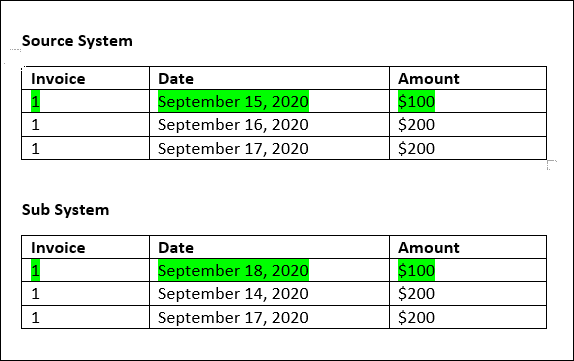4. Continuing to the second Source transaction (with a Date value of September 16), the first transaction from Sub System with a Date greater than or equal to September 16 and less than or equal to September 19 is found. This match of September 17 is within the range and identified in red below: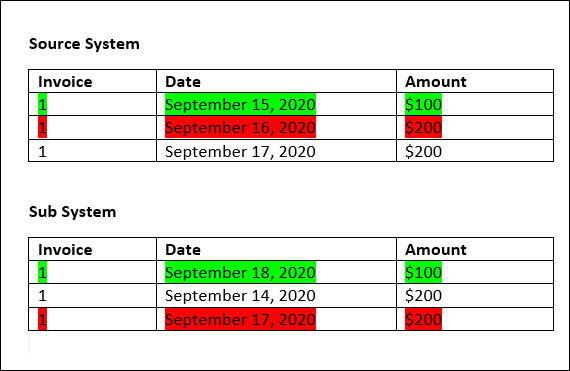5. Continuing with the third Source System transaction (with a Date value of September 17), assume we need to find the first transaction from Source System with a Date greater than or equal to September 16 and less than or equal to September 20. Since no transaction from Source System satisfies this condition, no match is created.

Note:

In the case of single source match processes, Positive transactions match to Negative transactions.

Evaluating 1 to Many Rule Types

With 1 to Many rule types, Source System transactions are the base transactions.

Note:

For 1 to Many rule types, you must define one rule condition which can be Match Exactly or with tolerance in addition to the Balancing Attribute.

Evaluating Many to 1 Rule Types

With Many to 1 rule types, Sub System transactions are the base transactions.

Note:

For Many to 1 rule types, you must define one rule condition which can be Match Exactly or with tolerance in addition to the Balancing attribute condition.

Evaluating Many to Many Rule Types

With Many to Many rule types, there is no base transaction. Rather, the conditions are evaluated using a set of rules.

The following process is used to evaluate a match for Many to Many rule types with Date tolerance specified.

1. Find the minimum date value and maximum date value from the source system.
2. From the subsystem, use the following formula to compute the minimum date value and the maximum date value that can be allowed when determining a match:
• Minimum subsystem date must be equal to or greater than maximum source system date + start value of the date tolerance range
• Maximum subsystem date must be equal to or less than minimum source system date + end value of the date tolerance range
3. Consider all subsystem transactions that are within the computed minimum subsystem date and maximum subsystem date when determining a match

Note that the minimum subsystem date must be equal to or less than the maximum subsystem date.

Example: Many to Many Rule Type with Date Tolerance

In this example, the date tolerance allowed for a match is -2 to +3.

Consider the following source system transactions for store ID 3738.

Table 8-1 Source System Transactions

Store ID Amount Date
3738 \$715 07-Feb-23
3738 \$595 07-Feb-23
3738 \$960 04-Feb-23
3738 -\$138 04-Feb-23

In the source system transactions, the minimum date value is 04-Feb-23 and the maximum date value is 07-Feb-23.

Consider the following subsystem transactions for store ID 3738.

Table 8-2 Subsystem Transactions

Store ID Amount Date
3738 \$387 05-Feb-23
3738 \$211 05-Feb-23
3738 \$378 07-Feb-23
3738 \$342 05-Feb-23
3738 \$714 06-Feb-23
3738 \$100 07-Feb-23

Using the formula in Step 2, determine the date range for subsystem transactions that must be considered for matching.

• Minimum subsystem date must be equal to or greater than 05-Feb-23 (07-Feb-23 - 2 days)
• Maximum subsystem date must be equal to or less than 07-Feb-23 (04-Feb-23 + 3 days).

Therefore, all subsystem transactions that are between 05-Feb-23 and 07-Feb-23 are considered while creating a match. In this example, all subsystem transactions fall within this range.

The sum of the Amounts in the source system is 2132. In the subsystem, the sum of Amounts of the transactions that are within the computed date range is 2132. Therefore, the source system and subsystem transactions are considered a match.

To understand an example when a match is not created, consider the same source system transactions shown above. In the subsystem transactions, the only change is that the last transaction (with Amount = 100) is dated 08-Feb-23. The maximum subsystem date that can be considered when creating a match is 07-Feb-23, so the transaction dated 08-Feb-23 is not included. The sum of Amounts in the source system is 2132, but the sum of Amounts in the subsystem is 2032. Therefore, these sets of source and subsystem transactions are not a match.

Note:

For Many to Many rules, you must define at least one Match Exactly condition.

Evaluating Match Rule Conditions for a Balancing Attribute

The method for evaluating match rule conditions for a Balancing Attribute is slightly different than for other attributes. Consider a Many to Many rule type using the sample data. Here's how the system evaluates this:
1. The system will first select all transactions with matching Invoice numbers (rule #1).
2. The Date attributes will then be evaluated to see if they satisfy the rule condition (rule #2).
3. Lastly, it will evaluate whether the Source System Amount matches exactly to the Sub System Amount (rule #3).

Since it’s a Many to Many match with multiple transactions in Source System and Sub System, the Amount values must first be summed by Data Source, and then the sum totals compared.

In our example, the sum of Source System Amount is \$500 and the sum of Sub System Amount is also \$500, so the match conditions are satisfied: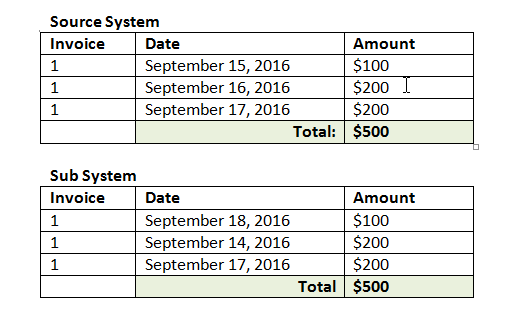Note:

The same process applies to 1 to Many matches and Many to 1 matches. Any time multiple transactions of the same data source exist for a match, the values of Balancing Attributes must first be summed before the comparison occurs.

Evaluating Tolerances Set as a Percentage and Variance Limit

The percentage tolerance option is available for Number and Integer type data source attributes (including balancing attribute) and can be set for:

• Auto match rules
• Manual match rule
• Default attribute mapping
Let's look at how matching works with tolerance low/high values as a percentage. For example, let's assume:
• Matching rule has 1% low and 1% high tolerance
• The tolerance on Up To Amount is set as .5
Source System Amount Sub System Amount Calculated Tolerance Variance (Source - Sub System Amt) Match Calculation Result
99.6 100 1% of 99.6 = .99 99.6 - 100 = .4 .4 < .99 and < .5 YES Match found
99.1 100 1% of 99.1 = .99 99.1 - 100 = .9 .9 < .99 but > .5 NO Match found since tolerance variance Up To limit was exceeded
100 99.6 1% of 100 = 1 100 - 99.6 = .4 .4 < 1 and < .5 YES Match Found
100 99.1 1% of 100 = 1 100 - 99.1 = .9 .9 < 1 and > .5 NO Match found since tolerance variance Up To limit was exceeded
50, 49.6 50, 50 1% of 99.6 = .99 99.6 - 100 = .4 .4 < .99 and < .5 YES Match Found

Evaluating Date Tolerances for 1 to 1 and 1 to Many Rules

The date tolerance is the number of days to consider for matching of transactions between two data sources. Transaction Matching considers date tolerance in the most common way of matching transactions, treating the Source (in the GL for example), as available "before" the Bank deposits or Sub System transactions. The engine anchors on the Source and calculates the range of date values to consider for matching forward from there based on the tolerance values entered in the rule.

The Date tolerance option is available for Date type data source attributes and can be set for:

• Auto match rules
• Manual match rule
• Default attribute mapping

Date tolerance is very flexible and you can use it to specify matching using a:

• Range of days

Use the date tolerance in rules to establish a range of days to consider for the matching. In rule creation, these fields are labelled Start and End to indicate the range. The engine provides a lot of flexibility for working with the date tolerance range. Let's say you want to consider transactions for matching between today and one day later. That would be Start and End date of 0 to 1. Another example is to start matching transactions two days from the Source transactions and ending 4 days out. You can also consider one day back (start as -1) to 1 day forward by entering a Start as -1 and End value of +1.

• Specific Date

Additional precision for the date tolerance is offered by being able to match transactions from one source to transactions in the Sub System on another specific day instead of a range of days. This is accomplished by using the date tolerance values in the Start and End fields as the same value. For example, let's say that you only want to match your Source transactions with the Bank transactions 2 days out. You can enter 2 in the Start field and 2 in the End field to only consider exactly that day for matching.

• Business Calendar instead of a regular calendar in order to eliminate holidays and weekends for consideration in matching.

By default, Account Reconciliation uses a regular calendar to specify the dates in a date range. You also have the option of using a business calendar when calculating date range in order to easily eliminate counting days that are work holidays for a company or weekends in calculation of the range. The Use Business Calendar check box is available as an option when creating Date Type attribute rule conditions with tolerance and also for match process default attribute mapping. The business calendar option is not available for manual matching.

Note:

To use a business calendar, you must specify the work days for your organizational unit as well as a holiday rule containing the list of holidays. The organizational units are assigned to profiles and the associated business calendar is then used during creation of reconciliations. See Defining Organizational Units in the Setting Up and Configuring Account Reconciliation guide.

Here is an example of a rule that has date tolerance specified as well as showing the business calendar checkbox.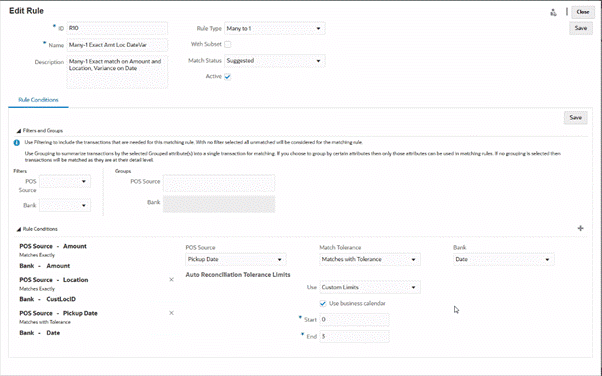For more details on creating rules with date tolerance when defining a match process, see Defining the Match Process by Creating Rules